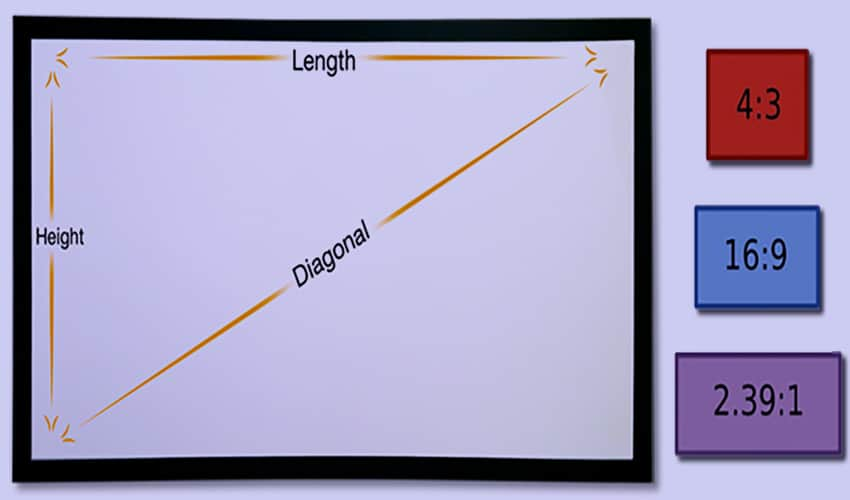# PPI To Pixels Converter

#### PPI To Pixels Converter

This is a free converter which you can use to convert pixels per inch (ppi) to pixels online quickly.

##### Aspect ratio (Ratio width and height):

:

PPI or Pixels per inch indicates the density of information (in pixels) for a given measurement (per inches).

Furthermore, the increase in ppi value not only indicates that the resolution is better but also indicates that you will get better quality images.

To convert ppi to pixels you should know your screen diagonal length in inches and the aspect ratio of your screen or display.

The size of a screen is often given by the length of its diagonal expressed in inches and the value ​​given by the manufacturers, whereas the aspect ratio of a screen represents the ratio between its length and its width.#### How To Use PPI To Pixels Converter

First, enter PPI value

Second, enter screen diagonal value in inches

Third, enter aspect ratio

Finally, click on convert button

#### How to calculate Pixels from PPI?

You can convert ppi to pixels using two methods, online method by the converter we provide above, in addition to manually method using the following three formulas:

First formula for calculating screen diagonal in pixels

Screen diagonal (pixels) = PPI * Screen diagonal (inches)

Second formula for calculating height in pixels

Height (pixels) = Screen diagonal (pixels)/√(Aspect ratio²+1)

Third formula for calculating width in pixels

Width (pixels) = Screen diagonal (pixels)/√((1/Aspect ratio²)+1)

Obviously the automatic online method using the converter we provide above is better in converting ppi to pixels than manually method, because it is fast, accurate and absolutely free.